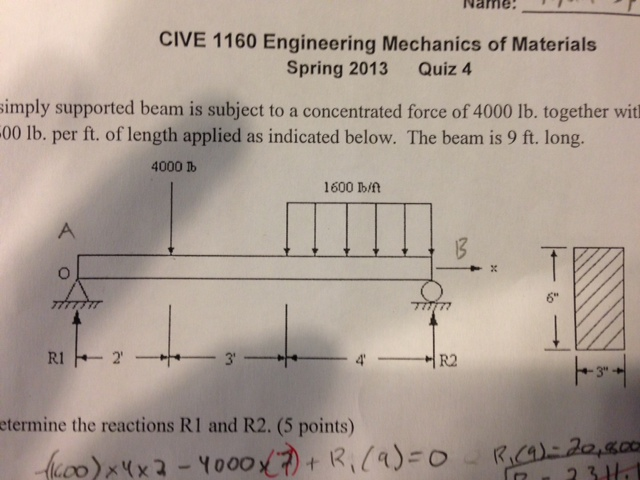# Question The simply supported beam is subject to a concentrated force of 4000 lb. together with a distributed load of 1600 lb per ft of length applied as indicated below. the beam is 9 ft long A)determine the reactions R1 and R2 B)Draw the shear force diagram and bending moment diagram C)find the maximum shear stress in this beam D) find the maximum bending stress in this beamCHEJ9F The Asker · Other EngineeringThe simply supported beam is subject to a concentrated force of 4000 lb. together with a distributed load of 1600 lb per ft of length applied as indicated below. the beam is 9 ft long

A)determine the reactions R1 and R2

B)Draw the shear force diagram and bending moment diagram

C)find the maximum shear stress in this beam

D) find the maximum bending stress in this beam

More